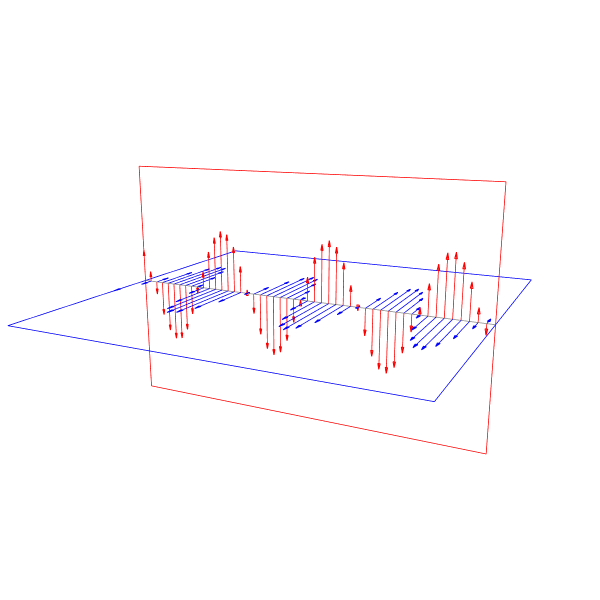# Plotting a vector field in a grid structure

Hello there, I’m solving the Maxwell’s equation numerically in R^3, and I’m trying to create a plot which draws a vector in the point in 3D space. Essentially, I’m trying to make a plot that looks like the following, minus the extra solid lines.

To put my code succinctly, I have two vectors, \vec{E}(x,y,z) and \vec{B}(x,y,z), and a grid in each spatial coordinate, where each component of the vector has a given value associated with it. In Julia, this is being represented by an N \times N \times N matrix, meaning that E_x, E_y and E_z is represented by an Array{Float64, 3} of size N \times N \times N, with the same taking place for vector \vec{B}.

What I would like to do was to issue a plot command that looked like,

vectorplot(xgrid, ygrid, zgrid, vector=(Ex, Ey, Ez))


with the code being made in such a way that for the point (xgrid, ygrid, zgrid) it would display a vector with components (Ex, Ex, Ex).

However, as far as I am aware, there is no such function. When using the Plots package I can make use of quiver to plot a vector field, but it’s arguments are of the type

quiver([x₀, x₁, ..., xₙ], [y₀, y₁, ..., yₙ], quiver=([a₀, a₁, ..., aₙ], [b₀, b₁, ..., bₙ]))


which plots vectors of size (a_i, b_i) with origin at (x_i, y_i). Even with other libraries, such as Gaston, the syntax is similar.

I could take the output of my simulation and change it accordingly to fit this syntax, however, it seems inefficient, given that this simulation might grow large, and, more frankly, I expect that there is a more ‘plug-n-play’ type of solution, developed by somebody who already faced the same issue that I am currently facing.

Could somebody provide me with some insight on what could be the best way to make such a plot happen?

Since there is no answer so far I try to give an idea I implemented when I needed a similar plot a few years ago. More precisely
I generated the following
animation in Python, with frames plotted as plotly.py figures.Here I post the Python code translated to Julia, to generate an unanimated plot, with Plots.jl.
My code does not implement your requirement to work with 3d arrays. It is just a code for this particular task.
I worked with 2d arrays (matrices).
One starts with the matrices start, enda1 and enda2 of size (n, 3). On each row are the coordinates
of starting points for arrows, respectively end points. enda1, respectively enda2
are end points for arrows within the plane of equation x=0, respectively z=0.
If S is the starting point for an arrow and E its end, then the unit vector of \vec{ES} multiplied by fract
gives the length of the head arrow. The head arrow can be controlled by its length and angle.
If \vec{u}=\text{fract}*\vec{ES}/||\vec{ES}, then v, w are the 2-vectors resulted by
rotating \vec{u} with π/10 (or another angle at your choice), respectively -π/10, and extended to 3-vectors in the plane x=0 or z=0.
A line connectting E->E+v->E+w->E defines a triangle, i.e. the arrow head.

using Plots
plotlyjs()
import LinearAlgebra: norm
import Rotations:RotMatrix

function arrowhead_dirs(arr_dir::Matrix{T}, arrow_angle::T; ids=1:2, fract=0.1) where T<:AbstractFloat
arr_dirm = mapreduce(permutedims, vcat, fract*[row/norm(row) for row in eachrow(arr_dir)])
v = RotMatrix{2}(arrow_angle)*arr_dirm[:, ids]'
w = RotMatrix{2}(-arrow_angle)*arr_dirm[:, ids]'
if ids ==1:2
return  vcat(v, zeros(size(v, 2))'), vcat(w, zeros(size(w, 2))')
elseif ids==2:3
return vcat(zeros(size(v, 2))', v), vcat(zeros(size(w, 2))', w)
else error("wrong ids =\$ids")
end
end

function get_arrows(start::Matrix{T}, enda::Matrix{T};
ids=1:2, fract=0.1, arrow_angle=pi/10) where T<:AbstractFloat
arr_dir=start-enda
v, w= arrowhead_dirs(arr_dir, arrow_angle; ids=ids, fract=fract)
p = enda+v'
q = enda+w'
#supp are  the lines segments as support for arrows
suppx, suppy, suppz = [hcat(start[:, i], enda[:, i], NaN*ones(size(start, 1) )) for i=1:3]
NaN*ones(size(enda, 1))) for i=1:3]
end

F(x; A=0.5, λ=0.7)=A*sin(x/λ)
b = 5
Y = -b:0.25:b
X = zeros(size(Y))
ZZe = zeros(size(Y))

start = hcat(X, Y, zeros(size(X)));
Ze = F.(Y;)
enda1 = hcat(X, Y, Ze)
x1, y1, z1, xx1, yy1, zz1 = get_arrows(start, enda1; ids=2:3)
#XXe = Ze
enda2 = hcat(Ze, Y, ZZe);
x2, y2, z2, xx2, yy2, zz2 = get_arrows(start, enda2; ids=1:2);

pl= plot(size=(600, 450), xaxis=false, yaxis=false, zaxis=false,
camera=(105, 25), legend=false)
plot!(pl, x1, y1, z1, c= :red, w=1)
plot!(pl, xx1, yy1, zz1, c= :red, w=2)
plot!(pl, x2, y2, z2, c= :blue, w=1)
plot!(pl, xx2, yy2, zz2, c= :blue, w=2)